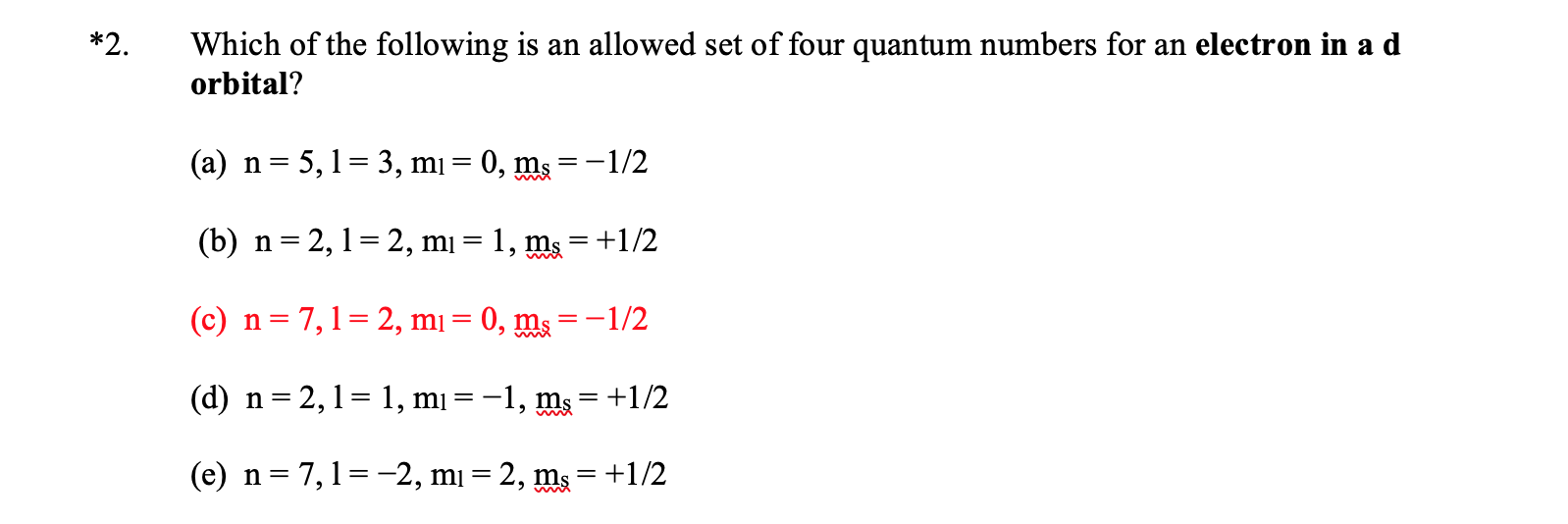# Which of the following is an allowed set of four quantum numbers for an electron in a d orbital? (a) n = 5, l = 3, ml = 0, ms = -1/2 (b) n = 2, l = 2, ml = 1, ms = +1/2 (c) n = 7, l = 2, ml = 0, ms = -1/2 (d) n = 2, l = 1, ml = -1, ms = +1/2 (e) n = 7, l = -2, ml = 2, ms = +1/2